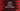# 3 different ways in Python to convert string to a tuple of integers## 3 different ways in Python to convert string to a tuple of integers:

One string with comma-separated integers is given like ‘1,2,3,4,5’, and we need to convert that string to a tuple of integers.

I will show you three different ways to solve this problem :

## Method 1: By using tuple, map and split :

The elements of the array are comma-separated. We can use split and map to split the items and get one map of integers. We can pass that map value to a constructor of tuple and it will convert that map to a tuple.

``````given_value = '1,2,3,4'
t = tuple(map(int, given_value.split(',')))

print("value of t: {}, and type: {}".format(t,type(t)))``````

In this program, we are printing the value of the tuple and its type.

It prints the below output :

``value of t: (1, 2, 3, 4), and type: <class 'tuple'>``

## Method 2: By using split, for..in and tuple :

Another way to solve this problem is by splitting the elements, iterating through the values and passing the values to a tuple constructor :

``````given_value = '1,2,3,4'
t = tuple(int(value) for value in given_value.split(','))

print("value of t: {}, and type: {}".format(t,type(t)))``````

We are printing the same values as the above example and it prints the same output.

## Method 3: By using eval :

This is the easiest way to solve this problem. eval converts a comma separated string of characters to a tuple.

``````given_value = '1,2,3,4'
t = eval(given_value)

print("value of t: {}, and type: {}".format(t,type(t)))``````

It prints the same output.